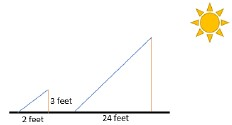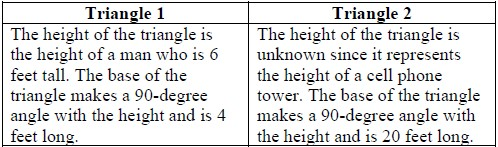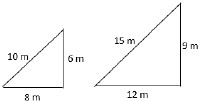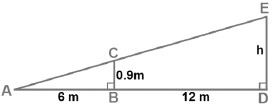# MA.8.GR.2.4Export Print
Solve mathematical and real-world problems involving proportional relationships between similar triangles.

### Examples

During a Tampa Bay Lightning game one player, Johnson, passes the puck to his teammate, Stamkos, by bouncing the puck off the wall of the rink. The path of the puck creates two line segments that form hypotenuses for each of two similar right triangles, with the height of each triangle the distance from one of the players to the wall of the rink. If Johnson is 12 feet from the wall and Stamkos is 3 feet from the wall. How far did the puck travel from the wall of the rink to Stamkos if the distance traveled from Johnson to the wall was 16 feet?
General Information
Subject Area: Mathematics (B.E.S.T.)
Strand: Geometric Reasoning
Status: State Board Approved

## Benchmark Instructional Guide

### Terms from the K-12 Glossary

• Proportional Relationships
• Similarity

### Vertical Alignment

Previous Benchmarks

Next Benchmarks

### Purpose and Instructional Strategies

In grade 7, students solved mathematical and real-world problems involving scale factors. In grade 8, students make connections of slope to the constant of proportionality through the use of similar triangle when represented in the coordinate plane (as stated in Clarification 2 in MA.8.AR.3.2). Additionally, students solve problems involving similar triangles. In high school, students will prove triangle congruence or similarity using Side-Side-Side, Side-Angle-Side, Angle-Side-Angle, Angle-Angle-Side, Angle-Angle and Hypotenuse-Leg.
• Instruction includes the definition of similarity applied to similar triangles, noting that the similar triangles will have the same shape but not necessarily the same size. This will extend to the angles being congruent and the sides being proportional.
• Students should be given real-world problem-solving opportunities in and out of the classroom to be able to visualize the work with similar triangles.
• For example, students should draw a picture to represent an application problem. If students were given the problem: If a tree casts a 24-foot shadow at the same time that a yardstick casts a 2-foot shadow, find the height of the tree. Students can draw triangles to represent the situation.### Common Misconceptions or Errors

• Students may incorrectly assume the sides and angles of similar triangles must be equivalent. To address this misconception, show a variety of examples of sizes of triangles that are similar.
• When checking to see if the triangles are proportional, students may incorrectly make connections to corresponding sides.
• For example, when triangles have been turned to a different orientation, students may incorrectly match the sides to check for proportionality.

### Strategies to Support Tiered Instruction

• Instruction includes co-creating foldables or graphic organizers with “Definition in Student’s Words, Examples, Non-Examples, and Real-World Application.” Present students with examples of triangles that are similar and of different sizes.
• Teacher provides objects that have the same shape and students label the corresponding sides.
• Teacher models use of geometric software to practice similarity and congruence.
• Instruction includes showing a variety of examples of sizes of triangles that are similar for students that incorrectly assume the sides and angles of a similar triangles must be equivalent.

Given the description of two similar triangles below, sketch each triangle described in the table.• Part A. What would be a reasonable height of a cell phone tower?
• Part B. Find the height of the cell phone tower. Is this a realistic height for the cell phone tower?
• Part C. Research heights of cell phone towers in your area. How does this answer compare to cell phone towers in your area?
• Part D. Using a typical height of cell phone towers in your area, what could you use in the real-world to create similar triangles to be able to calculate the height of your local cell phone tower?

### Instructional Items

Instructional Item 1
Given the two triangles, are they similar?Instructional Item 2
What is the height, $h$, in meters?*The strategies, tasks and items included in the B1G-M are examples and should not be considered comprehensive.

## Related Courses

This benchmark is part of these courses.
1205050: M/J Accelerated Mathematics Grade 7 (Specifically in versions: 2014 - 2015, 2015 - 2020, 2020 - 2022, 2022 and beyond (current))
1205070: M/J Grade 8 Pre-Algebra (Specifically in versions: 2014 - 2015, 2015 - 2022, 2022 and beyond (current))
1204000: M/J Foundational Skills in Mathematics 6-8 (Specifically in versions: 2014 - 2015, 2015 - 2022, 2022 and beyond (current))
7812030: Access M/J Grade 8 Pre-Algebra (Specifically in versions: 2014 - 2015, 2015 - 2018, 2018 - 2019, 2019 - 2022, 2022 and beyond (current))

## Related Access Points

Alternate version of this benchmark for students with significant cognitive disabilities.
MA.8.GR.2.AP.4: Use tools to solve mathematical problems using proportions between similar triangles.

## Related Resources

Vetted resources educators can use to teach the concepts and skills in this benchmark.

## Perspectives Video: Teaching Idea

Measuring Height with Triangles and Mirrors:

Reflect for a moment on how to measure tall objects with mirrors and mathematics.

Type: Perspectives Video: Teaching Idea

## Student Resources

Vetted resources students can use to learn the concepts and skills in this benchmark.

## Parent Resources

Vetted resources caregivers can use to help students learn the concepts and skills in this benchmark.